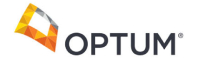New update is available. Click here to update.
Topics

# Level Order Traversal

Easy0/40
Average time to solve is 15m+54 more companies

## Problem statement

You have been given a Binary Tree of integers. You are supposed to return the level order traversal of the given tree.

For example:
``````For the given binary tree
````````````The level order traversal will be {1,2,3,4,5,6,7}.
``````
Detailed explanation ( Input/output format, Notes, Images )
Constraints:
``````1 <= T <= 100
0 <= N <= 1000
0 <= data <= 10^6 and data != -1
Where ‘T’ is the number of test cases, and ‘N’ is the total number of nodes in the binary tree, and “data” is the value of the binary tree node.

Time Limit: 1sec
``````
Sample Input 1:
``````3
1 2 3 4 -1 5 6 -1 7 -1 -1 -1 -1 -1 -1
1 2 3 -1 -1 -1 -1
1 3 -1 2 -1 -1 -1
``````
Sample Output 1:
``````1 2 3 4 5 6 7
1 2 3
1 3 2
``````
Explanation of Sample Input 1:
`````` For the first test case, {1} is at level 1 and {2,3} are at level 2 and {4,5,6} are at level 3 and {7} is at level 4. So combinations of all levels are {1, 2, 3 ,4, 5, 6, 7}.

For the second test case, {1} is at level 1 and {2,3} are at level 2. So combinations of level are {1, 2, 3}.

For the third test case, {1} is at level 1 and {3} is at level 2 and {2} is at level 3. So combinations of all levels are {1,3,2}.
``````
Sample Input 2:
``````2
2 7 5 2 6 -1 9 -1 -1 5 11 4 -1 -1 -1 -1 -1 -1 -1
1 2 3 4 -1 5 6 -1 -1 -1 -1 -1 -1
``````
Sample Output 2:
``````2 7 5 2 6 9 5 11 4
1 2 3 4 5 6
``````
Explanation of Sample Input 2:
``````For the first test case, {2} is at level 1 and {7,5} are at level 2 and {2,6,9} are at level 3 and {5,11,4} are at level 4. So combinations of all levels are {2, 7, 5, 2, 6, 9, 5, 11, 4}.

For the second test case, {1} is at level 1 and {2,3} are at level 2 and {4,5,6} are at level 3. So combinations of all levels are {1, 2, 3, 4, 5, 6}.
``````Console